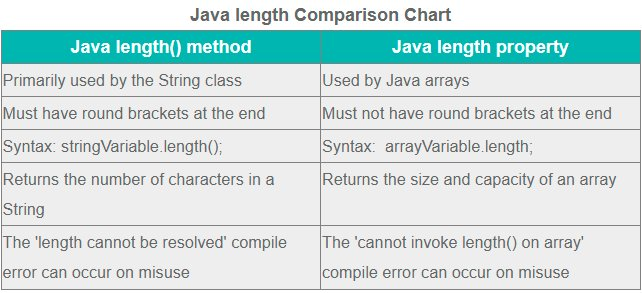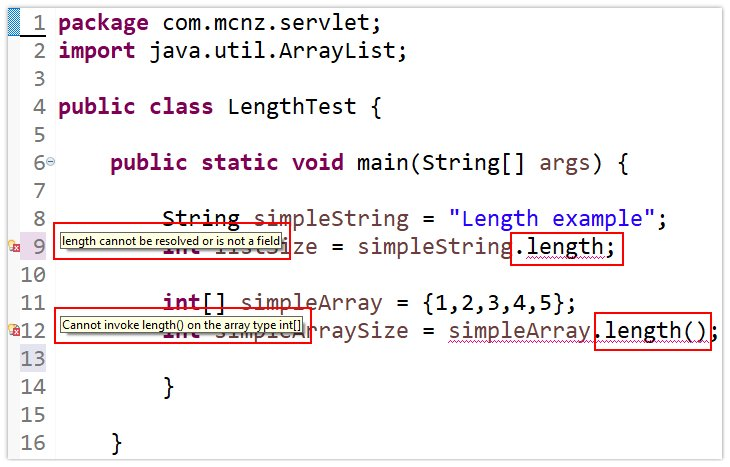# How do I find the Java array length?

## How to find the size of a Java array?

To find the size or length of a Java array, follow these four steps

1. Declare a variable of type array.
2. Initialize the Java array to a non-null value.
3. Use the length property of the array to get its size.
4. Hold the value of the Java array length in a variable for future use.

## The length property of a Java Array

Java arrays contain a length property that returns the array’s capacity.

The Java array’s length property does not return the number of non-null elements in the array. The Java array length represents the array’s total potential size, not just the number of elements that are in use.

```Array Class (Java 17)
length - returns the size of an array in terms
of its total capacity to hold elements```

## Code example to find the Java array size

Here is a simple example of how to find the length of a Java array in Java and then print the array’s size to the console:

```int[] myArray = {1,2,3,4,5};
int arraySize = myArray.length;
System.out.println(arraySize);
//The Java array length example prints out the number 5```

## Zero-based counting and array length

Arrays in Java use zero-based counting. This means that the first element in an array is at index zero.

However, the Java array length does not start counting at zero.

When you use the length property to find the size of an array that contains five elements, the value returned for the Java array length is five, not four.

## Java Array length vs String length()

New developers often confuse the Java array length property with the String class’ length() method. While their functions are similar, the syntax is different.

The Java array length property does not have round brackets after the field. The Java String length() method does. Confusion here will cause compile time errors, so be careful.Use the length property to find the size of a Java array. Do not treat the property as a method.

### Java array length compile errors

If you put round brackets on a Java array’s length method, the following compile time error will occur:

`Java Array Length Error: Cannot invoke length() on the array type int[]`

A NullPointer runtime exception error is also a possibility, if you try to find a Java array’s length but it isn’t initialized.To find the size of a Java array, make sure you invoke the length property, not the method.

## Using the Java array length for size

To determine the size of an array in your code, you need to use the Java array’s length property field.

Just make sure the array is not null, and don’t confuse Java’s length and length() concepts. Then you will have no problem working with the size and length of a Java array.

Close